×#### Thank you for registering.

One of our academic counsellors will contact you within 1 working day.

Click to Chat

1800-1023-196

+91-120-4616500

CART 0

• 0

MY CART (5)

Use Coupon: CART20 and get 20% off on all online Study Material

ITEM
DETAILS
MRP
DISCOUNT
FINAL PRICE
Total Price: Rs.

There are no items in this cart.
Continue Shopping• Complete JEE Main/Advanced Course and Test Series
• OFFERED PRICE: Rs. 15,900
• View Details

```Revision Notes on Direct and Inverse Proportions

Introduction to Direct and Inverse Proportions

There are so many situations in our life where we see some direct or indirect relationship between two things. Like

If the number of things purchased is increasing then the amount to pay will also increase.

If the speeds of the car will increase then the time to reach the destination will decreases.

Variations

If the value of two objects depends upon each other in such a way that the increase or decrease in the value of one object affects the value of another object then these two objects are said to be in variation.

Direct Proportion

Two quantities a and b are said to be in direct proportion if

Increase in a increases the b

Decrease in a decreases the b

But the ratio of their respective values must be the same.

a and b will be in direct proportion if a/b = k(k is constant) or a = kb.

In such a case if b1, b2 are the values of b corresponding to the values a1, a2 of a respectively then, a1/b2 = a2/b1​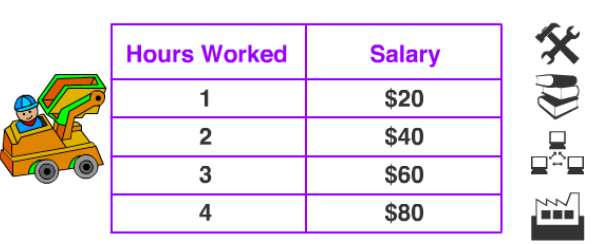This shows that as the no. of hours worked will increase the amount of salary will also increase with the constant ratio.

Symbol of ProportionWhen two quantities a and b are in proportion then they are written as a ∝ b where ∝ represents “is proportion to”.

Methods to solve Direct Proportion Problems

There are two methods to solve the problems related to direct proportion-

1. Tabular Method

As we know that,so, if one ratio is given then we can find the other values also. (The ratio remains the constant in the direct proportion).

Example

The cost of 4-litre milk is 200 Rs. Tabulate the cost of 2, 3, 5, 8 litres of milk of same quality.

Solution:

Let X litre of milk is of cost Y Rs.

X(Liter)
2
3
4
5
8

Y(Rupees)
Y2
Y3
200
Y4
Y5

We know that as the litre will increase the cost will also increase and if the litre will decrease then the cost will also decrease.

Given,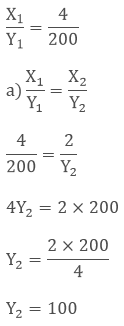So the cost of 2 ltr milk is 100 Rs.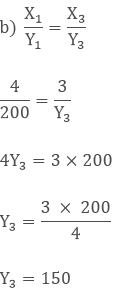So the cost of 3 ltr milk is 150 Rs.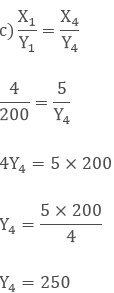So the cost of 5 ltr milk is 250 Rs.So the cost of 8 ltr milk is 400 Rs.

2. Unitary Method

If two quantities a and b are in direct proportion then the relation will be

k = a/b or a = kb

We can use this relation in solving the problem.

Example

If a worker gets 2000 Rs. to work for 4 hours then how much time will they work to get 60000 Rs.?

Solution:

Here,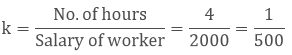By using this relation a = kb we can findHence, they have to work for 12 hours to get 60000 Rs.

Inverse Proportion

Two quantities a and b are said to be in Inverse proportion if

Increase in a decreases the b

Decrease in a increases the b

But the ratio of their respective values must be the same.

a and b will be in inverse proportion if k= ab

In such a case if b1, b2 are the values of b corresponding to the values a1, a2 of a respectively then, a1b1 = a2b2 = k,

When two quantities a and b are in inverse proportion then they are written as a ∝ 1/b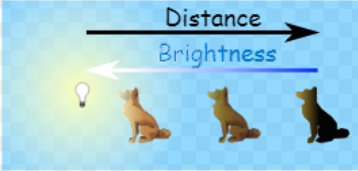This shows that as the distance of the figure from light increases then the brightness to the figure decreases.

Example

If 15 artists can make a statue in 48 hours then how many artists will be required to do the same work in 30 hours?

Solution:

Let the number of artists required to make a statue in 30 hours be y.

Number of hours
48
30

Number of artists
15
y

We know that as the no. of artists will increase the time to complete the work will reduce. So, the number of hours and number of artists are in inverse proportion.

So 48 × 15 = 30 × y (a1b1 = a2b2)

Therefore,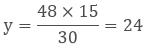So, 24 artists will be required to make the statue in 30 hours.
```### Course Features

• 728 Video Lectures
• Revision Notes
• Previous Year Papers
• Mind Map
• Study Planner
• NCERT Solutions
• Discussion Forum
• Test paper with Video Solution